Definitions of Square Dance Calls and Concepts

Index -->  Plus  |  A1  |  A2  |  C1  |  C2  |  C3A  |  C3B  |  C4  |  NOL  |
Definitions (Text Only) -->  Plus  |  A1  |  A2  |  C1  |  C2  |  C3A  |  C3B  |  C4  |  NOL  |
 Find call:

Criss Cross The Deucey [C3B]
(author unknown)

\$BJ?9T\$J(B Wave, \$BE,@Z\$J(B 2 x 4 T-Bone, \$B\$^\$?\$O(B All 4 Couples Touch 1/4 \$B\$N8e\$KF@\$i\$l\$k(B formation \$B\$+\$i(B.

Trailers \$B\$O(B Cross Extend, Arm Turn 3/4 and Spread \$B\$r\$7(B, Leaders \$B\$O(B 1/2 Split Circulate, Phantom Crossover Circulate \$B\$r\$7\$^\$9(B.

\$BJ?9T\$J(B Wave, Point-to-Point Diamonds, \$B\$^\$?\$O(B Thar \$B\$G=*\$o\$l\$^\$9(B.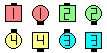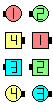Criss Cross The Deucey\$B\$NA0(B \$B8e(B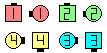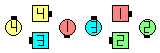Criss Cross The Deucey\$B\$NA0(B \$B8e(B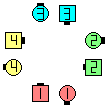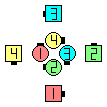Criss Cross The Deucey\$B\$NA0(B \$B8e(B

\$BCmCriss Cross The Deucey \$B\$O(B Criss Cross Your Neighbor [C2] \$B\$HF1\$8\$G\$9\$,(B, Leaders \$B\$O(B Cross Run \$B\$G\$J\$/(B Crossover Circulate \$B\$r\$7\$^\$9(B.

Criss Cross The Deucey \$B\$N(B Cheat: Weave & Trade The Deucey.

Criss Cross Your Neighbor [C2]: \$BJ?9T\$J(B Wave, \$BE,@Z\$J(B 2 x 4 T-Bone \$B\$+\$i(B. Trailers \$B\$O(B Cross Extend, Arm Turn 3/4 (Cross Your Neighbor) & Spread \$B\$r\$7(B, Leaders \$B\$O(B 1/2 Box Circulate & Cross Run \$B\$r\$7\$^\$9(B. Mini Wave \$B\$N(B Box \$B\$+\$i\$O(B, \$B5U\$NCriss Cross The Diamond [C4]: \$BJ?9T\$J(B Wave \$B\$+\$i(B. Criss Cross The Deucey; Centers Hinge; Diamond Circulate; Centers Hinge. \$BJ?9T\$J(B Wave \$B\$G=*\$o\$j\$^\$9(B. (Cheat: Weave & Trade The Diamond.)Choreography for Criss Cross The DeuceyComments? Questions? Suggestions?

https://www.ceder.net/def/crisscrossthedeucey.php?language=japan
01-July-2022 03:52:03